Hostname: page-component-cd4964975-xtmlv Total loading time: 0 Render date: 2023-03-30T15:54:54.416Z Has data issue: true Feature Flags: { "useRatesEcommerce": false } hasContentIssue true

# Mid-IR cosmological spectrophotometric surveys from space: Measuring AGN and star formation at the cosmic noon with a SPICA-like mission

Published online by Cambridge University Press:  23 April 2021

*
Author for correspondence: Luigi Spinoglio, E-mail: luigi.spinoglio@inaf.it

## Abstract

We use the SPace Infrared telescope for Cosmology and Astrophysics (SPICA) project as a template to demonstrate how deep spectrophotometric surveys covering large cosmological volumes over extended fields (1–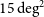$15\, \rm{deg^2}$ ) with a mid-IR imaging spectrometer (17–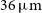$36\, \rm{\rm{\upmu m}}$ ) in conjunction with deep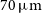$70\, \rm{\rm{\upmu m}}$ photometry with a far-IR camera, at wavelengths which are not affected by dust extinction can answer the most crucial questions in current galaxy evolution studies. A SPICA-like mission will be able for the first time to provide an unobscured three-dimensional (3D, i.e. x, y, and redshift z) view of galaxy evolution back to an age of the universe of less than$\sim$ 2 Gyrs, in the mid-IR rest frame. This survey strategy will produce a full census of the Star Formation Rate (SFR) in the universe, using polycyclic aromatic hydrocarbons (PAH) bands and fine-structure ionic lines, reaching the characteristic knee of the galaxy luminosity function, where the bulk of the population is distributed, at any redshift up to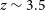$z \sim 3.5$ . Deep follow-up pointed spectroscopic observations with grating spectrometers onboard the satellite, across the full IR spectral range (17–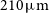$210\, \rm{\rm{\upmu m}}$ ), would simultaneously measure Black Hole Accretion Rate (BHAR), from high-ionisation fine-structure lines, and SFR, from PAH and low- to mid-ionisation lines in thousands of galaxies from solar to low metallicities, down to the knee of their luminosity functions. The analysis of the resulting atlas of IR spectra will reveal the physical processes at play in evolving galaxies across cosmic time, especially its heavily dust-embedded phase during the activity peak at the cosmic noon (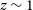$z \sim 1$ –3), through IR emission lines and features that are insensitive to the dust obscuration.

Type
Research Article
© The Author(s), 2021. Published by Cambridge University Press on behalf of the Astronomical Society of Australia

## Access options

Get access to the full version of this content by using one of the access options below. (Log in options will check for institutional or personal access. Content may require purchase if you do not have access.)

## References

Alonso-Herrero, A., Pereira-Santaella, M., Rieke, G. H., & Rigopoulou, D. 2012, ApJ, 744, 2Google Scholar
Amorín, R. O., Pérez-Montero, E., & Vílchez, J. M. 2010, ApJ, 715, L128Google Scholar
André, P., et al. 2019, PASA, 36, e029Google Scholar
Aoyama, S., Hou, K.-C., Hirashita, H., Nagamine, K., & Shimizu, I. 2018, MNRAS, 478, 4905Google Scholar
Armus, L., et al. 2007, ApJ, 656, 148CrossRefGoogle Scholar
Behroozi, P., Wechsler, R. H., Hearin, A. P., & Conroy, C. 2019, MNRAS, 488, 3143Google Scholar
Bernard-Salas, J., et al. 2009, ApJS, 184, 230Google Scholar
Bianchi, S., & Schneider, R. 2007, MNRAS, 378, 973Google Scholar
Blanton, M. R., & Berlind, A. A. 2007, ApJ, 664, 791CrossRefGoogle Scholar
Bocchio, M., Jones, A. P., & Slavin, J. D. 2014, A&A, 570, A32Google Scholar
Bocchio, M., Marassi, S., Schneider, R., Bianchi, S., Limongi, M., & Chieffi, A. 2016, A&A, 587, A157Google Scholar
Bond, J. R., Kofman, L., & Pogosyan, D. 1996, Natur, 380, 603Google Scholar
Bouwens, R. J., et al. 2015, ApJ, 803, 34Google Scholar
Brandl, B. R., et al. 2006, ApJ, 653, 1129Google Scholar
Bundy, K., et al. 2015, ApJ, 798, 7Google Scholar
Calura, F., Pipino, A., & Matteucci, F. 2008, A&A, 479, 669Google Scholar
Carpenter, J., Iono, D., Kemper, F., & Wootten, A. 2020, arXiv e-prints, p.Google Scholar
Ceccarelli, C., Viti, S., Balucani, N., & Taquet, V. 2018, MNRAS, 476, 1371CrossRefGoogle Scholar
Cicone, C., et al. 2014, A&A, 562, A21Google Scholar
Ciesla, L., et al. 2015, A&A, 576, A10CrossRefGoogle Scholar
Comastri, A., Gilli, R., Marconi, A., Risaliti, G., & Salvati, M. 2015, A&A, 574, L10Google Scholar
Cormier, D., et al. 2015, A&A, 578, A53Google Scholar
Cormier, D., et al. 2019, A&A, 626, A23Google Scholar
Cortzen, I., et al. 2019, MNRAS, 482, 1618CrossRefGoogle Scholar
Cucciati, O., et al. 2012, A&A, 539, A31Google Scholar
Cullen, F., et al. 2019, MNRAS, 487, 2038Google Scholar
Dasyra, K. M., Ho, L. C., Netzer, H., Combes, F., Trakhtenbrot, B., Sturm, E., Armus, L., & Elbaz, D. 2011, ApJ, 740, 94Google Scholar
de Graauw, T., et al. 1996, A&A, 315, L49Google Scholar
De Looze, I., Barlow, M. J., Swinyard, B. M., Rho, J., Gomez, H. L., Matsuura, M., & Wesson, R. 2017, MNRAS, 465, 3309Google Scholar
Delvecchio, I., et al. 2014, MNRAS, 439, 2736Google Scholar
Diamond-Stanic, A. M., & Rieke, G. H. 2012, ApJ, 746, 168CrossRefGoogle Scholar
Dors, O. L., et al. 2013, MNRAS, 432, 2512Google Scholar
Draine, B. T., Li, A., Hensley, B. S., Hunt, L. K., Sandstrom, K., & Smith, J. D. T. 2020, arXiv e-prints, p.Google Scholar
Duras, F., et al. 2020, A&A, 636, A73Google Scholar
Elbaz, D., et al. 2007, A&A, 468, 33Google Scholar
Elbaz, D., et al. 2011, A&A, 533, A119Google Scholar
Fernández-Ontiveros, J. A., Spinoglio, L., Pereira-Santaella, M., Malkan, M. A., Andreani, P., & Dasyra, K. M. 2016, ApJS, 226, 19Google Scholar
Fernández-Ontiveros, J. A., et al. 2017, PASA, 34, e053Google Scholar
Ferrara, A., Viti, S., & Ceccarelli, C. 2016, MNRAS, 463, L112CrossRefGoogle Scholar
Fiore, F., et al. 2017, A&A, 601, A143Google Scholar
Franco, M., et al. 2018, A&A, 620, A152Google Scholar
Gallimore, J. F., et al. 2010, ApJS, 187, 172CrossRefGoogle Scholar
Gardner, J. P., et al. 2006, SSR, 123, 485Google Scholar
Gatkine, P., Vogel, S., & Veilleux, S. 2020, ApJ, 897, 9CrossRefGoogle Scholar
Georgantopoulos, I., & Akylas, A. 2019, A&A, 621, A28CrossRefGoogle Scholar
Gilli, R., Comastri, A., & Hasinger, G. 2007, A&A, 463, 79CrossRefGoogle Scholar
Gjergo, E., Granato, G. L., Murante, G., Ragone-Figueroa, C., Tornatore, L., & Borgani, S. 2018, MNRAS, 479, 2588CrossRefGoogle Scholar
González-Alfonso, E., et al. 2017a, PASA, 34, e054Google Scholar
González-Alfonso, E., et al. 2017b, ApJ, 836, 11Google Scholar
Goulding, A. D., & Alexander, D. M. 2009, MNRAS, 398, 1165CrossRefGoogle Scholar
Goulding, A. D., Alexander, D. M., Bauer, F. E., Forman, W. R., Hickox, R. C., Jones, C., Mullaney, J. R., & Trichas, M. 2012, ApJ, 755, 5CrossRefGoogle Scholar
Graziani, L., Schneider, R., Ginolfi, M., Hunt, L. K., Maio, U., Glatzle, M., & Ciardi, B. 2020, MNRAS, 494, 1071CrossRefGoogle Scholar
Green, J., et al. 2012, arXiv e-prints, p.Google Scholar
Greiner, J., et al. 2015, ApJ, 809, 76CrossRefGoogle Scholar
Griffin, M. J., et al. 2010, A&A, 518, L3Google Scholar
Gruppioni, C., et al. 2013, MNRAS, 432, 23Google Scholar
Gruppioni, C., et al. 2016, MNRAS, 458, 4297Google Scholar
Gruppioni, C., et al. 2017, PASA, 34, e055Google Scholar
Gruppioni, C., et al. 2020, A&A, 643, A8Google Scholar
Heckman, T. M., & Best, P. N. 2014, ARA&A, 52, 589Google Scholar
Herrera-Camus, R., et al. 2018, ApJ, 861, 95Google Scholar
Hirashita, H. 2012, MNRAS, 422, 1263Google Scholar
Ho, L. C., & Keto, E. 2007, ApJ, 658, 314CrossRefGoogle Scholar
Hollenbach, D. J., & Tielens, A. G. G. M. 1997, ARA&A, 35, 179CrossRefGoogle Scholar
Houck, J. R., et al. 2004, ApJS, 154, 18Google Scholar
Hunt, L., Dayal, P., Magrini, L., & Ferrara, A. 2016, MNRAS, 463, 2002CrossRefGoogle Scholar
Imanishi, M., Ichikawa, K., Takeuchi, T., Kawakatu, N., Oi, N., & Imase, K. 2011, PASJ, 63, 447CrossRefGoogle Scholar
IveziĆ, Ž., et al. 2019, ApJ, 873, 111Google Scholar
Kaneda, H., et al. 2016, in Space Telescopes and Instrumentation 2016: Optical, Infrared, and Millimeter Wave. p. 99042I, doi: 10.1117/12.2232442 Google Scholar
Kaneda, H., et al. 2017, PASA, 34, e059Google Scholar
Kashino, D., et al. 2019, ApJS, 241, 10Google Scholar
Kennicutt, R. C., & Evans, N. J. 2012, ARA&A, 50, 531Google Scholar
Kessler, M. F., et al. 1996, A&A, 500, 493Google Scholar
Kirkpatrick, A., et al. 2017, ApJ, 849, 111Google Scholar
Kormendy, J., & Ho, L. C. 2013, ARA&A, 51, 511Google Scholar
La Caria, M. M., Vignali, C., Lanzuisi, G., Gruppioni, C., & Pozzi, F. 2019, MNRAS, 487, 1662CrossRefGoogle Scholar
Lambrides, E. L., Chiaberge, M., Heckman, T., Gilli, R., Vito, F., & Norman, C. 2020, ApJ, 897, 160CrossRefGoogle Scholar
Lapi, A., et al.2011, ApJ, 742, 24Google Scholar
Laporte, N., et al. 2017, ApJ, 837, L21CrossRefGoogle Scholar
Laureijs, R., et al. 2011, arXiv e-prints, p.Google Scholar
Le Floc’h, E., et al. 2005, ApJ, 632, 169CrossRefGoogle Scholar
Lilly, S. J., Tresse, L., Hammer, F., Crampton, D., & Le Fevre, O. 1995, ApJ, 455, 108Google Scholar
Luridiana, V., Morisset, C., & Shaw, R. A. 2015, A&A, 573, A42Google Scholar
Lusso, E., et al. 2012, MNRAS, 425, 623Google Scholar
Lutz, D., Kunze, D., Spoon, H. W. W., & Thornley, M. D. 1998, A&A, 333, L75Google Scholar
Lutz, D., Sturm, E., Genzel, R., Moorwood, A. F. M., Alexander, T., Netzer, H., & Sternberg, A. 2000, ApJ, 536, 697Google Scholar
Lutz, D., et al. 2008, ApJ, 684, 853Google Scholar
Madau, P., Ferguson, H. C., Dickinson, M. E., Giavalisco, M., Steidel, C. C., & Fruchter, A. 1996, MNRAS, 283, 1388Google Scholar
Magnelli, B., et al. 2013, A&A, 553, A132Google Scholar
Maiolino, R., et al. 2008, A&A, 488, 463Google Scholar
Małek, K., Buat, V., Burgarella, D., Roehlly, Y., Shirley, R., & Team, HELP 2020, in IAU Proceedings, Vol. 341, Panchromatic Modelling with Next Generation Facilities, ed. M. Boquien, E. Lusso, C. Gruppioni, & P. Tissera, 39 (), doi: 10.1017/S174392131900471X Google Scholar
Mancini, M., Schneider, R., Graziani, L., Valiante, R., Dayal, P., Maio, U., Ciardi, B., & Hunt, L. K. 2015, MNRAS, 451, L70Google Scholar
Mannucci, F., et al. 2009, MNRAS, 398, 1915Google Scholar
Marassi, S., Schneider, R., Limongi, M., Chieffi, A., Graziani, L., & Bianchi, S. 2019, MNRAS, 484, 2587CrossRefGoogle Scholar
Matsuura, M., et al. 2019, MNRAS, 482, 1715Google Scholar
McKinney, J., Pope, A., Armus, L., Chary, R.-R., Díaz-Santos, T., Dickinson, M. E., & Kirkpatrick, A. 2020, ApJ, 892, 119CrossRefGoogle Scholar
McKinnon, R., Torrey, P., Vogelsberger, M., Hayward, C. C., & Marinacci, F. 2017, MNRAS, 468, 1505Google Scholar
Meixner, M., et al. 2019, arXiv e-prints, p.Google Scholar
Murphy, E. J., Chary, R. R., Dickinson, M., Pope, A., Frayer, D. T., & Lin, L. 2011, ApJ, 732, 126Google Scholar
Nakagawa, T., Shibai, H., Onaka, T., Matsuhara, H., Kaneda, H., Kawakatsu, Y., & Roelfsema, P. 2014, in Space Telescopes and Instrumentation 2014: Optical, Infrared, and Millimeter Wave. p. 91431I, doi: 10.1117/12.2055947 CrossRefGoogle Scholar
Nandra, K., et al. 2013, arXiv e-prints, p.Google Scholar
Nardini, E., Risaliti, G., Watabe, Y., Salvati, M., & Sani, E. 2010, MNRAS, 405, 2505Google Scholar
Negrello, M., et al. 2017, MNRAS, 470, 2253CrossRefGoogle Scholar
Neugebauer, G., et al. 1984, ApJ, 278, L1Google Scholar
Noeske, K. G., et al. 2007, ApJ, 660, L47CrossRefGoogle Scholar
Oliver, S. J., et al. 2012, MNRAS, 424, 1614Google Scholar
Onodera, M., et al. 2016, ApJ, 822, 42CrossRefGoogle Scholar
Pereira-Santaella, M., Diamond-Stanic, A. M., Alonso-Herrero, A., & Rieke, G. H. 2010, ApJ, 725, 2270CrossRefGoogle Scholar
Pérez-González, P. G., et al. 2005, ApJ, 630, 82Google Scholar
Pérez-González, P. G., et al. 2008, ApJ, 675, 234Google Scholar
Petric, A. O., et al. 2011, ApJ, 730, 28CrossRefGoogle Scholar
Pilbratt, G. L., et al. 2010, A&A, 518, L1CrossRefGoogle Scholar
Planck, Collaboration, et al. 2016, A&A, 594, A13Google Scholar
Poglitsch, A., et al. 2010, A&A, 518, L2Google Scholar
Puget, J. L., & Leger, A. 1989, ARA&A, 27, 161CrossRefGoogle Scholar
Reddy, N. A., Pettini, M., Steidel, C. C., Shapley, A. E., Erb, D. K., & Law, D. R. 2012, ApJ, 754, 25CrossRefGoogle Scholar
Rieke, G. H., et al. 2004, ApJS, 154, 25Google Scholar
Rigby, J. R., Diamond-Stanic, A. M., & Aniano, G. 2009, ApJ, 700, 1878Google Scholar
Rodighiero, G., et al. 2010a, A&A, 515, A8Google Scholar
Rodighiero, G., et al. 2010b, A&A, 518, L25Google Scholar
Rodighiero, G., et al. 2011, ApJ, 739, L40CrossRefGoogle Scholar
Roelfsema, P. R., et al. 2018, PASA, 35, e030Google Scholar
Runco, J. N., Malkan, M. A., Fernández-Ontiveros, J. A., Spinoglio, L., & Pereira-Santaella, M. 2020, ApJ, 905, 57Google Scholar
Rush, B., Malkan, M. A., & Spinoglio, L. 1993, ApJS, 89, 1CrossRefGoogle Scholar
Sanders, D. B., & Mirabel, I. F. 1996, ARA&A, 34, 749CrossRefGoogle Scholar
Sanders, D. B., Mazzarella, J. M., Kim, D. C., Surace, J. A., & Soifer, B. T. 2003, AJ, 126, 1607CrossRefGoogle Scholar
Sanders, R. L., et al. 2020a, arXiv e-prints, p.Google Scholar
Sanders, R. L., et al. 2020b, MNRAS, 491, 1427Google Scholar
Santini, P., et al. 2010, A&A, 518, L154Google Scholar
Sarangi, A., & Cherchneff, I. 2015, A&A, 575, A95Google Scholar
Schreiber, C., et al. 2015, A&A, 575, A74Google Scholar
Scoville, N., et al. 2007, ApJS, 172, 38Google Scholar
Scoville, N., et al., 2017, ApJ, 837, 150CrossRefGoogle Scholar
Shipley, H. V., Papovich, C., Rieke, G. H., Brown, M. J. I., & Moustakas, J. 2016, ApJ, 818, 60CrossRefGoogle Scholar
Shirley, R., et al. 2019, MNRAS, 490, 634Google Scholar
Sibthorpe, B., Helmich, F., Roelfsema, P., Kaneda, H., & Shibai, H. 2015, in EAS Publications Series, 411, doi: 10.1051/eas/1575083 CrossRefGoogle Scholar
Silk, J., & Mamon, G. A. 2012, RAA, 12, 917CrossRefGoogle Scholar
Smith, J. D. T., et al. 2007, ApJ, 656, 770Google Scholar
Somerville, R. S., & Davé, R. 2015, ARA&A, 53, 51CrossRefGoogle Scholar
Spinoglio, L., Dasyra, K. M., Franceschini, A., Gruppioni, C., Valiante, E., & Isaak, K. 2012, ApJ, 745, 171CrossRefGoogle Scholar
Spinoglio, L., Dasyra, K. M., Franceschini, A., Gruppioni, C., Valiante, E., & Isaak, K. 2014, ApJ, 791, 138Google Scholar
Spinoglio, L., & Malkan, M. A. 1992, ApJ, 399, 504CrossRefGoogle Scholar
Spinoglio, L., Malkan, M. A., Smith, H. A., González-Alfonso, E., & Fischer, J. 2005, ApJ, 623, 123Google Scholar
Spinoglio, L., et al. 2017, PASA, 34, e057Google Scholar
Spoon, H. W. W., & Holt, J. 2009, ApJ, 702, L42Google Scholar
Spoon, H. W. W., et al. 2013, ApJ, 775, 127CrossRefGoogle Scholar
Springel, V., et al. 2005, Nature, 435, 629CrossRefGoogle Scholar
Steidel, C. C., Strom, A. L., Pettini, M., Rudie, G. C., Reddy, N. A., & Trainor, R. F. 2016, ApJ, 826, 159CrossRefGoogle Scholar
Sturm, E., Lutz, D., Verma, A., Netzer, H., Sternberg, A., Moorwood, A. F. M., Oliva, E., & Genzel, R. 2002, A&A, 393, 821CrossRefGoogle Scholar
Sunyaev, R. A., & Zeldovich, Y. B. 1972, A&A, 20, 189Google Scholar
Suzuki, T. L., et al. 2020, arXiv e-prints, p.Google Scholar
Swinyard, B., et al. 2009, EA, 23, 193Google Scholar
Tacchella, S., Dekel, A., Carollo, C. M., Ceverino, D., DeGraf, C., Lapiner, S., Mand elker, N., & Primack Joel, R. 2016, MNRAS, 457, 2790Google Scholar
Thomas, D., Greggio, L., & Bender, R. 1999, MNRAS, 302, 537CrossRefGoogle Scholar
Todini, P., & Ferrara, A. 2001, MNRAS, 325, 726CrossRefGoogle Scholar
Tombesi, F., Meléndez, M., Veilleux, S., Reeves, J. N., González-Alfonso, E., & Reynolds, C. S. 2015, Natur, 519, 436CrossRefGoogle Scholar
Tommasin, S., Spinoglio, L., Malkan, M. A., & Fazio, G. 2010, ApJ, 709, 1257Google Scholar
Tommasin, S., Spinoglio, L., Malkan, M. A., Smith, H., González-Alfonso, E., & Charmand aris, V. 2008, ApJ, 676, 836CrossRefGoogle Scholar
Torrey, P., et al. 2019, MNRAS, 484, 5587Google Scholar
Treister, E., Urry, C. M., & Virani, S. 2009, ApJ, 696, 110CrossRefGoogle Scholar
Troncoso, P., et al. 2014, A&A, 563, A58CrossRefGoogle Scholar
Tsai, J. C., & Mathews, W. G. 1995, ApJ, 448, 84CrossRefGoogle Scholar
Ueda, Y., Akiyama, M., Hasinger, G., Miyaji, T., & Watson, M. G. 2014, ApJ, 786, 104CrossRefGoogle Scholar
Umehata, H., et al. 2019, Science, 366, 97CrossRefGoogle Scholar
Vaccari, M. 2016, in The Universe of Digital Sky, Vol. 42, ed. N. R. Surveys, Napolitano, G. Longo, M. Marconi, M. Paolillo, & E. Iodice, 71 (), doi: 10.1007/978-3-319-19330-4_10 CrossRefGoogle Scholar
Valiante, R., Schneider, R., Salvadori, S., & Bianchi, S. 2011, MNRAS, 416, 1916CrossRefGoogle Scholar
Vasudevan, R. V., & Fabian, A. C. 2009, MNRAS, 392, 1124CrossRefGoogle Scholar
Vergani, S. D., et al. 2015, A&A, 581, A102Google Scholar
Vermeij, R., & van der Hulst, J. M. 2002, A&A, 391, 1081CrossRefGoogle Scholar
Vogelsberger, M., et al. 2014, MNRAS, 444, 1518CrossRefGoogle Scholar
Wang, T., et al. 2019a, Natur, 572, 211Google Scholar
Wang, L., Pearson, W. J., Cowley, W., Trayford, J. W., Béthermin, M., Gruppioni, C., Hurley, P., & MichaŁowski, M. J. 2019b, A&A, 624, A98CrossRefGoogle Scholar
Wechsler, R. H., & Tinker, J. L. 2018, ARA&A, 56, 435CrossRefGoogle Scholar
Werner, M. W., et al. 2004, ApJS, 154, 1Google Scholar
White, S. D. M., & Rees, M. J. 1978, MNRAS, 183, 341CrossRefGoogle Scholar
Wootten, A., & Thompson, A. R. 2009, IEEE Proceedings, 97, 1463CrossRefGoogle Scholar
Wu, H., Cao, C., Hao, C.-N., Liu, F.-S., Wang, J.-L., Xia, X.-Y., Deng, Z.-G., & Young, C. K.-S. 2005, ApJ, 632, L79CrossRefGoogle Scholar
Wuyts, E., et al. 2014, ApJ, 789, L40CrossRefGoogle Scholar
Xie, Y., & Ho, L. C. 2019, ApJ, 884, 136CrossRefGoogle Scholar
Xie, Y., Li, A., & Hao, L. 2017, ApJS, 228, 6CrossRefGoogle Scholar
Yamaguchi, Y., et al. 2019, ApJ, 878, 73Google Scholar
Zhuang, M.-Y., Ho, L. C., & Shangguan, J. 2019, ApJ, 873, 103CrossRefGoogle Scholar